# Case Based Questions Test: Real Numbers - 2

## 10 Questions MCQ Test NCERT Mathematics for CAT Preparation | Case Based Questions Test: Real Numbers - 2

Description
Attempt Case Based Questions Test: Real Numbers - 2 | 10 questions in 20 minutes | Mock test for Class 10 preparation | Free important questions MCQ to study NCERT Mathematics for CAT Preparation for Class 10 Exam | Download free PDF with solutions
QUESTION: 1

### Direction: Read the following text and answer the following questions on the basis of the same:A seminar is being conducted by an Educational Organisation, where the participants will be educators of different subjects. The number of participants in Hindi, English and Mathematics are 60, 84 and 108 respectively.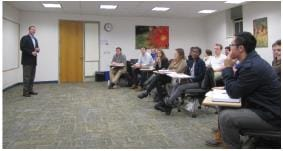108 can be expressed as a product of its primes as

Solution: All even numbers except 2 are composite numbers, so 108 is a composite number.

Therefore, we can conclude that 108 can be expressed as a product of prime factors by 22 × 33.

QUESTION: 2

### Direction: Read the following text and answer the following questions on the basis of the same:A seminar is being conducted by an Educational Organisation, where the participants will be educators of different subjects. The number of participants in Hindi, English and Mathematics are 60, 84 and 108 respectively.What is the minimum number of rooms required during the event?

Solution: Minimum no. of rooms required are total number of students divided by number of students in each room.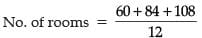= 21

QUESTION: 3

### Direction: Read the following text and answer the following questions on the basis of the same:A seminar is being conducted by an Educational Organisation, where the participants will be educators of different subjects. The number of participants in Hindi, English and Mathematics are 60, 84 and 108 respectively.The product of HCF and LCM of 60,84 and 108 is

Solution: 60 = (22 × 3 × 5)

84 = (22 × 3 × 7)

108 = (22 × 33)

HCF(60, 84,108) = (22 × 3) = 12

LCM =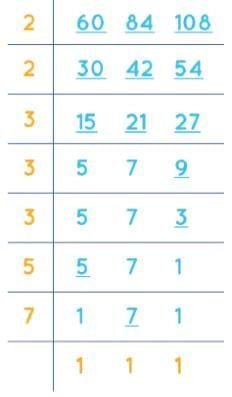LCM of 60, 84, and 108 is 3780.

Product of HCF x LCM = 3780 x 12 = 45360

QUESTION: 4

Direction: Read the following text and answer the following questions on the basis of the same:

A seminar is being conducted by an Educational Organisation, where the participants will be educators of different subjects. The number of participants in Hindi, English and Mathematics are 60, 84 and 108 respectively.In each room the same number of participants are to be seated and all of them being in the same subject, hence maximum number participants that can accommodated in each room are

Solution: No. of participants seated in each room would be HCF of all the three values above.

60 = 2 × 2 × 3 × 5

84 = 2 × 2 × 3 × 7

108 = 2 × 2 × 3 × 3 × 3

Hence, HCF = 12.

QUESTION: 5

Direction: Read the following text and answer the following questions on the basis of the same:

A seminar is being conducted by an Educational Organisation, where the participants will be educators of different subjects. The number of participants in Hindi, English and Mathematics are 60, 84 and 108 respectively.The LCM of 60, 84 and 108 is

Solution: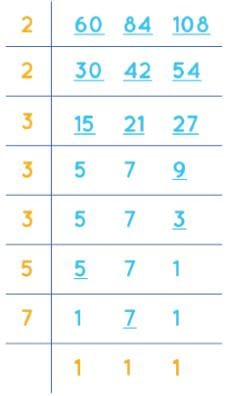LCM = 2 x 2 x 3 x 3 x 3 x 5 x 7

LCM = 3780

Therefore, LCM of 60, 84, and 108 is 3780.

QUESTION: 6

Direction: Read the following text and answer the following questions on the basis of the same:

A Mathematics Exhibition is being conducted in your School and one of your friends is making a model of a factor tree. He has some difficulty and asks for your help in completing a quiz for the audience.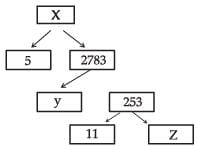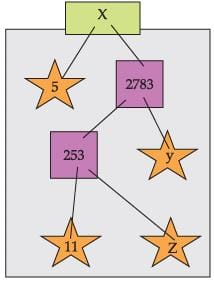The prime factorisation of 13915 is

Solution:

Using the prime factorization tree, we have

∴ 13915 = 5 × 11 × 11 × 23 = 5 × 112 × 23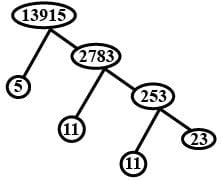QUESTION: 7

Direction: Read the following text and answer the following questions on the basis of the same:

A Mathematics Exhibition is being conducted in your School and one of your friends is making a model of a factor tree. He has some difficulty and asks for your help in completing a quiz for the audience.What will be the value of y?

Solution: 2783 = y × 253

y = 2783/253

y = 11

QUESTION: 8

Direction: Read the following text and answer the following questions on the basis of the same:

A Mathematics Exhibition is being conducted in your School and one of your friends is making a model of a factor tree. He has some difficulty and asks for your help in completing a quiz for the audience.According to Fundamental Theorem of Arithmetic 13915 is a

Solution: The prime factorization of 13,915 is 5 × 112 × 23.

Since it has a total of 4 prime factors, 13,915 is a composite number.

QUESTION: 9

Direction: Read the following text and answer the following questions on the basis of the same:

A Mathematics Exhibition is being conducted in your School and one of your friends is making a model of a factor tree. He has some difficulty and asks for your help in completing a quiz for the audience.What will be the value of x?

Solution: x = 2783 × 5

x = 13915

QUESTION: 10

Direction: Read the following text and answer the following questions on the basis of the same:

A Mathematics Exhibition is being conducted in your School and one of your friends is making a model of a factor tree. He has some difficulty and asks for your help in completing a quiz for the audience.What will be the value of z?

Solution: 253 = 11 × z

z = 253/11

z = 23Use Code STAYHOME200 and get INR 200 additional OFF Use Coupon Code